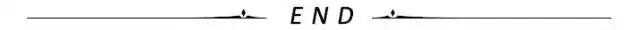# 深度讲解Python四大常用绘图库的“绘图原理”

2020/07/28 00:06

## 1. matplotlib绘图原理

matplotlib绘图原理：http://suo.im/678FCo

#### 1）绘图原理说明

• ① 导库;
• ② 创建 figure 画布对象;
• ③ 获取对应位置的 axes 坐标系对象;
• ④ 调用axes对象，进行对应位置的图形绘制;
• ⑤ 显示图形;

#### 2）案例说明

# 1.导入相关库import matplotlib as mplimport matplotlib.pyplot as plt# 2.创建figure画布对象figure = plt.figure()# 3.获取对应位置的axes坐标系对象axes1 = figure.add_subplot(2,1,1)axes2 = figure.add_subplot(2,1,2)# 4.调用axes对象，进行对应位置的图形绘制axes1.plot([1,3,5,7],[4,9,6,8])axes2.plot([1,2,4,5],[8,4,6,2])# 5.显示图形figure.show()

## 2. seaborn绘图原理

seaborn是matplotlib的更高级的封装。因此学习seaborn之前，首先要知道matplotlib的绘图原理。由于seaborn是matplotlib的更高级的封装，对于matplotlib的那些调优参数设置，也都可以在使用seaborn绘制图形之后使用。

seaborn绘图原理：http://suo.im/5D3VPX

#### 1）案例说明

# 1.导入相关库import seaborn as snsimport matplotlib.pyplot as pltdf = pd.read_excel("data.xlsx",sheet_name="数据源")sns.set_style("dark")plt.rcParams["font.sans-serif"] = ["SimHei"]plt.rcParams["axes.unicode_minus"] = False# 注意：estimator表示对分组后的销售数量求和。默认是求均值。sns.barplot(x="品牌",y="销售数量",data=df,color="steelblue",orient="v",estimator=sum)plt.show()

注意：可以看到在上述的绘图代码中，你应该有这样一个感受，图中既有matplotlib的绘图代码，也有seaborn的绘图代码。其实就是这样的，我们就是按照matplobt的绘图原理进行图形绘制，只是有些地方改成seaborn特有的代码即可，剩下的调整格式，都可以使用matplotlib中的方法进行调整。

## 3. plotly绘图原理

• plotly是一个基于javascript的绘图库，plotly绘图种类丰富，效果美观；
• 易于保存与分享plotly的绘图结果，并且可以与Web无缝集成；
• ploty默认的绘图结果，是一个HTML网页文件，通过浏览器可以直接查看；

plotly绘图原理：http://suo.im/5vxNTu

#### 1）绘图原理说明

• ① 绘制图形轨迹，在ployly里面叫做 trace ，每一个轨迹是一个trace。
• ② 将轨迹包裹成一个列表，形成一个“轨迹列表”。一个轨迹放在一个列表中，多个轨迹也是放在一个列表中。
• ③ 创建画布的同时，并将上述的 轨迹列表 ，传入到 Figure() 中。
• ④ 使用 Layout() 添加其他的绘图参数，完善图形。
• ⑤ 展示图形。

#### 2）案例说明

import numpy as npimport pandas as pdimport plotly as pyimport plotly.graph_objs as goimport plotly.expression as pxfrom plotly import toolsdf = pd.read_excel("plot.xlsx")# 1.绘制图形轨迹，在ployly里面叫做trace，每一个轨迹是一个trace。trace0 = go.Scatter(x=df["年份"],y=df["城镇居民"],name="城镇居民")trace1 = go.Scatter(x=df["年份"],y=df["农村居民"],name="农村居民")# 2.将轨迹包裹成一个列表，形成一个“轨迹列表”。一个轨迹放在一个列表中，多个轨迹也是放在一个列表中。data = [trace0,trace1]# 3.创建画布的同时，并将上述的轨迹列表，传入到Figure()中。fig = go.Figure(data)# 4.使用Layout()添加其他的绘图参数，完善图形。fig.update_layout(    title="城乡居民家庭人均收入",    xaxis_title="年份",    yaxis_title="人均收入（元）")# 5.展示图形。fig.show()

## 4. pyecharts绘图原理

Echarts是一个由百度开源的数据可视化工具，凭借着良好的交互性，精巧的图表设计，得到了众多开发者的认可。而python是一门富有表达力的语言，很适合用于数据处理。当数据分析遇上了数据可视化时，pyecharts诞生了。

pyecharts分为v0.5v1两个大版本，v0.5和v1两个版本不兼容，v1是一个全新的版本，因此我们的学习尽量都是基于v1版本进行操作。

pyecharts的绘图原理：http://suo.im/5S1PF1

#### 1）绘图原理说明

• ① 选择图表类型；
• ② 声明图形类并添加数据；
• ③ 选择全局变量；
• ④ 显示及保存图表；

#### 2）案例说明

# 1.选择图表类型：我们使用的是线图，就直接从charts模块中导入Line这个模块；from pyecharts.charts import Lineimport pyecharts.options as optsimport numpy as npx = np.linspace(0,2 * np.pi,100)y = np.sin(x)( # 2.我们绘制的是Line线图，就需要实例化这个图形类，直接Line()即可； Line() # 3.添加数据，分别给x,y轴添加数据； .add_xaxis(xaxis_data=x) .add_yaxis(series_name="绘制线图",y_axis=y,label_opts=opts.LabelOpts(is_show=False)) .set_global_opts(title_opts=opts.TitleOpts(title="我是标题",subtitle="我是副标题",title_link="https://www.baidu.com/"),                  tooltip_opts=opts.TooltipOpts())).render_notebook() # 4.render_notebook()用于显示及保存图表；

## 小结0
4 收藏

### 作者的其它热门文章0 评论
4 收藏
0